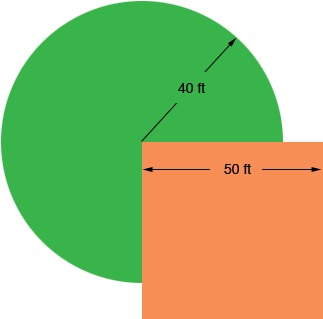Topic: A goat is tied to the corner of a 50 ft square outbuilding with a 40 ft. rope. a) What is the measure of the partial circumference created as the goat walks at the full length of the rope? b) Since the goat is trimming the grass from part of the outbuilding, how much of the perimeter of the outbuilding will the building owner have to trim? If it's okay can you show me how you got your answer and the formulas that were used. thank you for your time. Danielle 7th grade student is asking the question Hi Danielle, I drew a diagram. The green region is where the goat can graze and the beige region is the outbuilding.The partial circumference where the goat can graze is three quarters of the circumference of a circle of radius 40 ft. Do you know the circumference of a circle of radius 40 ft? The part of the perimeter of the outbuilding that is inside the goats grazing area is two sections of length 40 ft each. How much of the perimeter of the outbuilding is left? Penny